Next: Cavities with Rectangular Boundaries Up: Resonant Cavities and Waveguides Previous: Introduction

# Boundary Conditions

The general boundary conditions on the field vectors at an interface between medium 1 and medium 2 (say) are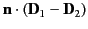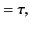(1294)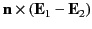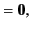(1295)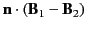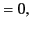(1296)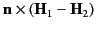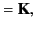(1297)

where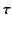is used for the interfacial surface change density (to avoid confusion with the conductivity), and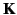is the surface current density. Here,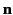is a unit vector normal to the interface, directed from medium 2 to medium 1. We saw in Section 7.4 that, at normal incidence, the amplitude of an electromagnetic wave falls off very rapidly with distance inside the surface of a good conductor. In the limit of perfect conductivity (i.e.,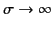), the wave does not penetrate into the conductor at all, in which case the internal tangential electric and magnetic fields vanish. This implies, from Equations (1297) and (1299), that the tangential component of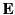vanishes just outside the surface of a good conductor, whereas the tangential component of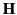may remain finite. Let us examine the behavior of the normal field components.

Let medium 1 be a conductor, of conductivity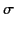and dielectric constant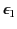, for which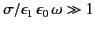, and let medium 2 be a perfect insulator of dielectric constant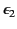. The change density that forms at the interface between the two media is related to the currents flowing inside the conductor. In fact, the conservation of charge requires that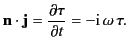(1298)

However,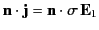, so it follows from Equation (1296) that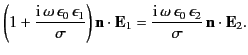(1299)

Thus, it is clear that the normal component ofwithin the conductor also becomes vanishingly small as the conductivity approaches infinity.

Ifvanishes inside a perfect conductor then the curl ofalso vanishes, and the time rate of change of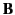is correspondingly zero. This implies that there are no oscillatory fields whatever inside such a conductor, and that the fields just outside satisfy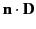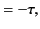(1300)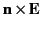(1301)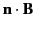(1302)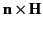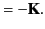(1303)

Here,is a unit normal at the surface of the conductor pointing into the conductor. Thus, the electric field is normal, and the magnetic field tangential, at the surface of a perfect conductor. For good conductors, these boundary conditions yield excellent representations of the geometrical configurations of the external fields, but they lead to the neglect of some important features of real fields, such as losses in cavities and signal attenuation in waveguides.

In order to estimate such losses, it is helpful to examine how the tangential and normal fields compare whenis large but finite. Equations (773) and (808) imply that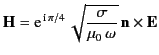(1304)

at the surface of a good conductor (provided that the wave propagates into the conductor). Let us assume, without obtaining a complete solution, that a wave withvery nearly tangential andvery nearly normal propagates parallel to the surface of a good conductor. According to the Faraday-Maxwell equation,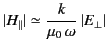(1305)

just outside the surface, where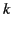is the component of the wavevector parallel to the surface. However, Equation (1306) implies that a tangential component ofis accompanied by a small tangential component of. By comparing the previous two expressions, we obtain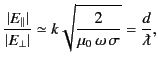(1306)

where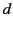is the skin depth [see Equation (810)] and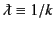. It is clear that the ratio of the tangential to the normal component ofis of order the skin depth divided by the wavelength. It is readily demonstrated that the ratio of the normal to the tangential component ofis of the same order of magnitude. Thus, we deduce that, in the limit of high conductivity, which implies vanishing skin depth, no fields penetrate into the conductor, and the boundary conditions are those given by Equations (1302)-(1305). Let us investigate the solution of the homogeneous wave equation subject to such constraints.Next: Cavities with Rectangular Boundaries Up: Resonant Cavities and Waveguides Previous: Introduction
Richard Fitzpatrick 2014-06-27